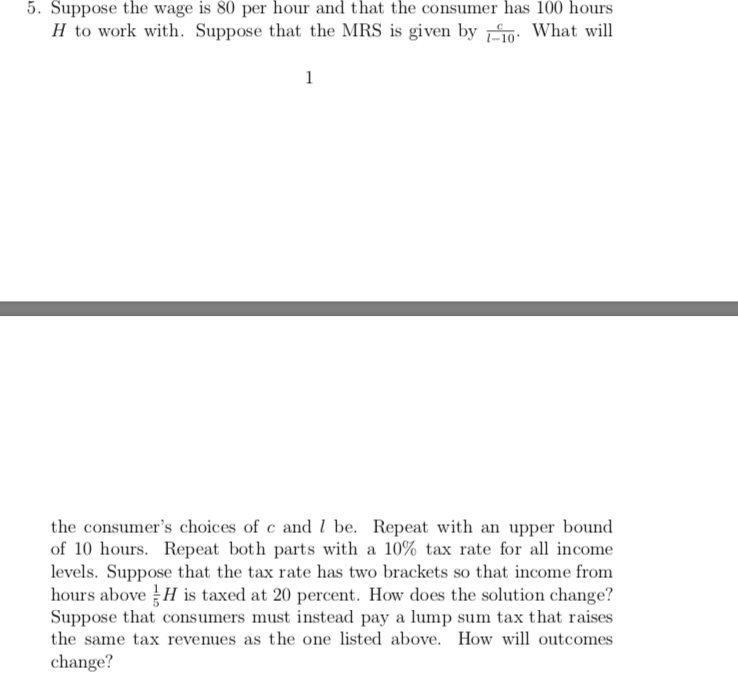# 5. Suppose the wage is 80 per hour and that the consumer has 100 hoursH to work with. Suppose that the MRS is given by in: What will1the consumer's choices of c and I be. Repeat with an upper boundof 10 hours. Repeat both parts with a 10% tax rate for all incomelevels. Suppose that the tax rate has two brackets so that income fromhours above H is taxed at 20 percent. How does the solution change?Suppose that consumers must instead pay a lump sum tax that raisesthe same tax revenues as the one listed above. How will outcomeschange?

Question
114 viewshelp_outlineImage Transcriptionclose5. Suppose the wage is 80 per hour and that the consumer has 100 hours H to work with. Suppose that the MRS is given by in: What will 1 the consumer's choices of c and I be. Repeat with an upper bound of 10 hours. Repeat both parts with a 10% tax rate for all income levels. Suppose that the tax rate has two brackets so that income from hours above H is taxed at 20 percent. How does the solution change? Suppose that consumers must instead pay a lump sum tax that raises the same tax revenues as the one listed above. How will outcomes change? fullscreen
check_circle

Step 1

The budget constraint of the individual can be written as follows:

Step 2

The equation policy is that the MRS is equal to which means that at the equation, the MRS equals wages equals is 100, C equals to 100l. The budget constraint of the economy can be expressed as follows:

Step 3

Thus, the value of ‘l’ c...

### Want to see the full answer?

See Solution

#### Want to see this answer and more?

Solutions are written by subject experts who are available 24/7. Questions are typically answered within 1 hour.*

See Solution
*Response times may vary by subject and question.
Tagged in